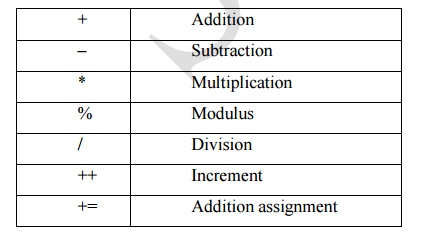Home | JAVA - Arithmetic Operators

# JAVA - Arithmetic Operators

Arithmetic operators are used in mathematical expressions in the same way that they are used in algebra. The following table lists the arithmetic operators:

Arithmetic Operators

Arithmetic operators are used in mathematical expressions in the same way that they are used in algebra. The following table lists the arithmetic operators:â€“        Subtraction

*        Multiplication

%      Modulus

/         Division

++     Increment

â€“=     Subtraction assignment

*=      Multiplication assignment

%=    Modulus assignment

/=      Division assignment

â€“ â€“     Decrement

The Bitwise Operators

Java defines several bitwise operators that can be applied to the integer types, long, int, short, char, and byte. These operators act upon the individual bits of their operands. They are summarized in the following table:

Operator     Result

~        Bitwise unary NOT

&       Bitwise AND

|         Bitwise OR

^        Bitwise exclusive OR

>>     Shift right

>>>   Shift right zero fill

<<     Shift left

&=    Bitwise AND assignment

|=       Bitwise OR assignment

^=      Bitwise exclusive OR assignment

>>=   Shift right assignment

>>>= Shift right zero fill assignment

<<=   Shift left assignment

Study Material, Lecturing Notes, Assignment, Reference, Wiki description explanation, brief detail
Web or internet Programming : JAVA : JAVA - Arithmetic Operators |